Question

A firm that is in the 35% tax bracket forecasts that it can retain \$3 million of new earnings plans to raise new capital in the following proportions:

 50% from 20-year bonds with a flotation cost of 5% of face value. Their current bonds are selling at a price of 92 (92% of face value), have 5 years remaining, have an annual coupon of 7.2%, and their investment bank thinks that new bonds will have a 50 basis point (0.50%) higher yield-to-maturity than their current 5 year bonds due to their longer term. 10% from preferred stock with a flotation cost of 6% of face value. The firm currently has an outstanding issue of \$50 face value fixed-rate preferred stock with an annual dividend of \$3 per share, and the stock is currently selling at \$36 per share. Any newly issued preferred stock will continue with the \$50 par-value, and will continue the \$3 dividend. 40% from equity. Their common dividend payout ratio is 60%, they recently paid a dividend of \$1.60 per share, the dividend is expected to grow to \$3.50 in 10 years, and is expected to continue this growth rate into the foreseeable future. The common stock has a current market price of \$19, and their investment banker suggests a flotation cost of 6% of market value on new common equity.

The after-tax cost of the new bond financing is 7.2%

The after-tax cost of the new preferred stock financing is 9.09%

The after-tax cost of retained earnings financing is 17.25%

The after-tax cost of the new common equity financing is 17.83%

The company's WACC using retained earnings as the source of equity is 11.41%

The break point in the cost of capital schedule due to running out of retained earnings is \$7.5 million

Part 7: Calculate the company's WACC after it substitutes the new common stock issue for retained earnings after it runs out of retained earnings. _________

Part 8: (Optional) Draw the cost of capital schedule for the firm. This schedule does not need to be elaborate.

We need at least 10 more requests to produce the answer.

0 / 10 have requested this problem solution

The more requests, the faster the answer.

All students who have requested the answer will be notified once they are available.

#### Earn Coins

Coins can be redeemed for fabulous gifts.

Similar Homework Help Questions
• ### A firm that is in the 35% tax bracket forecasts that it can retain \$4 million...

A firm that is in the 35% tax bracket forecasts that it can retain \$4 million of new earnings plans to raise new capital in the following proportions: 60% from 30-year bonds with a flotation cost of 4% of face value. Their current bonds are selling at a price of 91 (91% of face value), have 4 years remaining, have an annual coupon of 7%, and their investment bank thinks that new bonds will have a 40 basis point (0.40%)...

• ### A firm that is in the 35% tax bracket forecasts that it can retain \$4 million...

A firm that is in the 35% tax bracket forecasts that it can retain \$4 million of new earnings plans to raise new capital in the following proportions: 60% from 30-year bonds with a flotation cost of 4% of face value. Their current bonds are selling at a price of 91 (91% of face value), have 4 years remaining, have an annual coupon of 7%, and their investment bank thinks that new bonds will have a 40 basis point (0.40%)...

• ### Calculation of individual costs and WACC   Lang Enterprises is interested in measuring its overall cost of capital. Current investigation has gathered the following data. The firm is in the 30​% tax b...

Calculation of individual costs and WACC   Lang Enterprises is interested in measuring its overall cost of capital. Current investigation has gathered the following data. The firm is in the 30​% tax bracket. Debt  The firm can raise debt by selling ​\$1,000​-par-value, 7​% coupon interest​ rate, 16​-year bonds on which annual interest payments will be made. To sell the​ issue, an average discount of ​\$20 per bond would have to be given. The firm also must pay flotation costs of ​\$25...

• ### Turnbull Co. has a target capital structure of 58% debt, 6% preferred stock, and 36% common equity. It has a before-tax...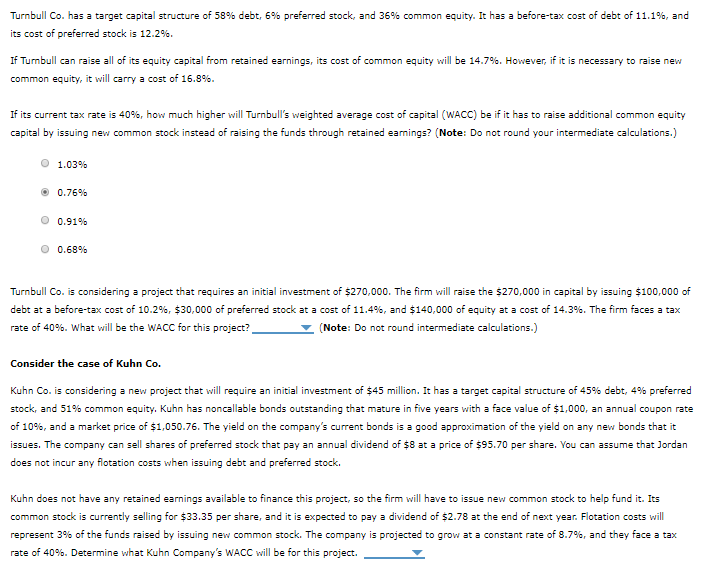Turnbull Co. has a target capital structure of 58% debt, 6% preferred stock, and 36% common equity. It has a before-tax cost of debt of 11.1%, and its cost of preferred stock is 12.2%. If Turnbull can raise all of its equity capital from retained earnings, its cost of common equity will be 14.7%. However, if it is necessary to raise new common equity, it will carry a cost of 16.8%. If its current tax rate is 40%, how much...

• ### Turnbull Co. has a target capital structure of 58% debt, If its current tax rate is...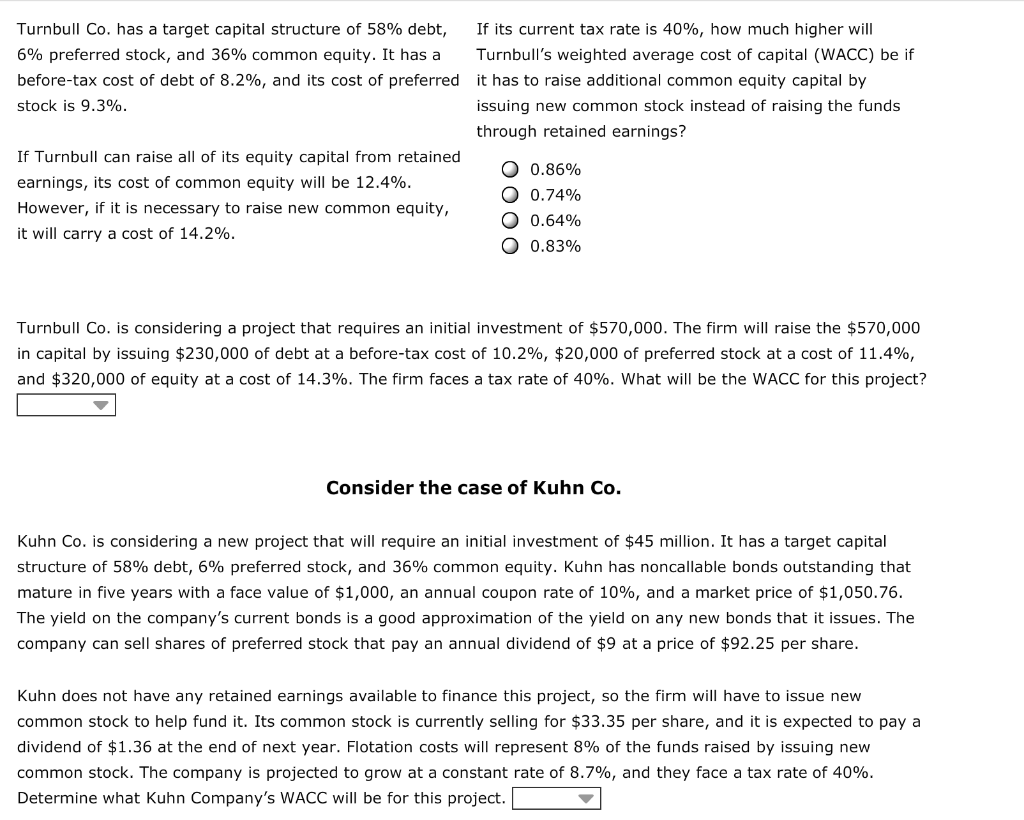Turnbull Co. has a target capital structure of 58% debt, If its current tax rate is 40%, how much higher will 6% preferred stock, and 36% common equity. It has a Turnbull's weighted average cost of capital (WACC) be if before-tax cost of debt of 8.2%, and its cost of preferred it has to raise additional common equity capital by stock is 9.3%. issuing new common stock instead of raising the funds through retained earnings? If Turnbull can raise all...

• ### a.  The​ after-tax cost of debt using the​ bond's yield to maturity​ (YTM) is The​ after-tax...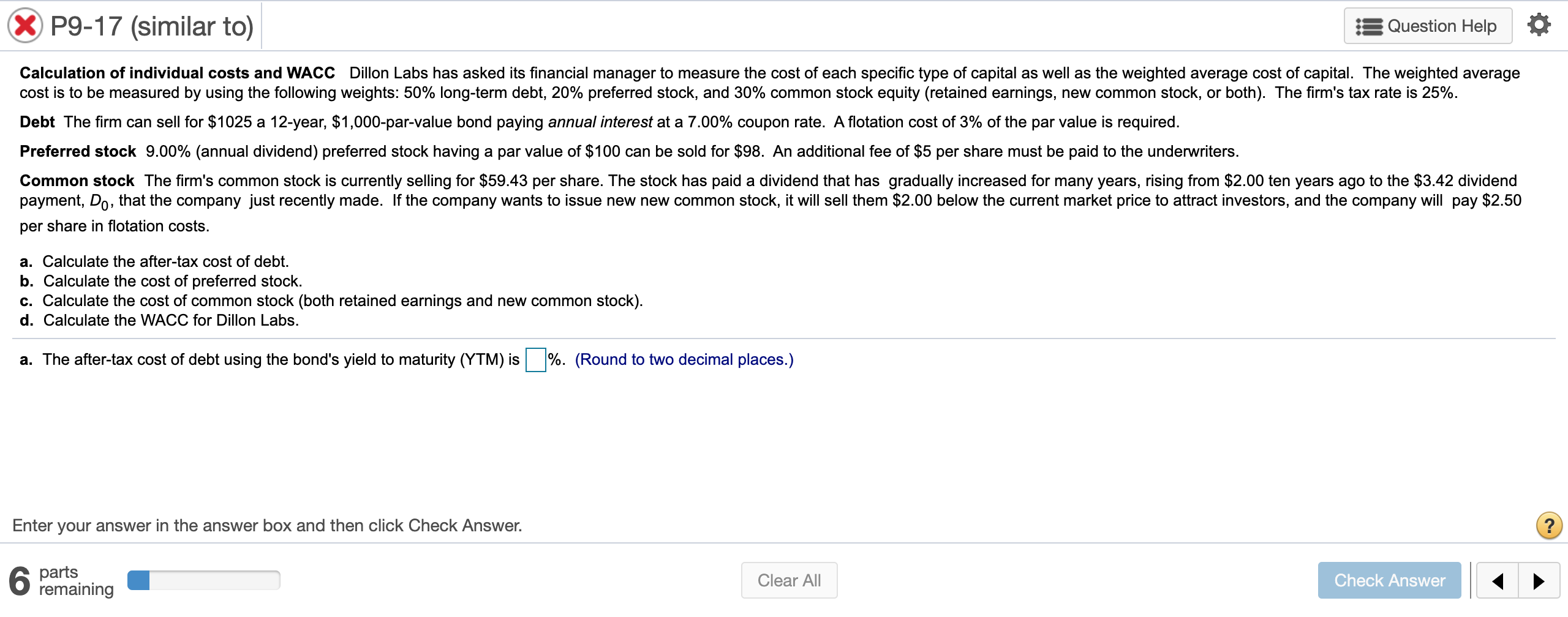a.  The​ after-tax cost of debt using the​ bond's yield to maturity​ (YTM) is The​ after-tax cost of debt using the approximation formula is b.  The cost of preferred stock is c.  The cost of retained earnings is The cost of new common stock is d.  Using the cost of retained​ earnings, the​ firm's WACC is Using the cost of new common​ stock, the​ firm's WACC is X P9-17 (similar to) Question Help Calculation of individual costs and WACC Dillon...

• ### Calculation of individual costs and WACC   Lang Enterprises is interested in measuring its overall cost of...

Calculation of individual costs and WACC   Lang Enterprises is interested in measuring its overall cost of capital. Current investigation has gathered the following data. The firm is in the 30​% tax bracket. Debt  The firm can raise debt by selling ​\$1,000​-par-value, 7​% coupon interest​ rate, 16​-year bonds on which annual interest payments will be made. To sell the​ issue, an average discount of ​\$20 per bond would have to be given. The firm also must pay flotation costs of ​\$25...

• ### Can someone help me understand this You develop the following information. Your firm has a target...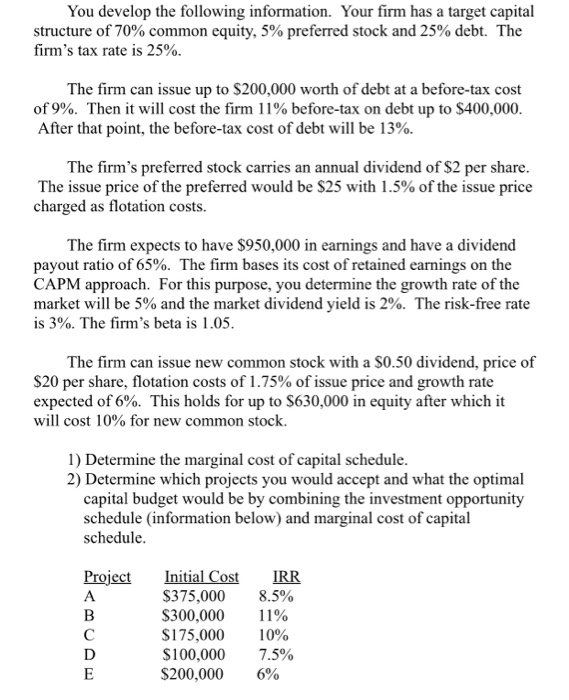Can someone help me understand this You develop the following information. Your firm has a target capital structure of 70% common equity, 5% preferred stock and 25% debt. The firm's tax rate is 25%. The firm can issue up to \$200,000 worth of debt at a before-tax cost of 9%. Then it will cost the firm 11% before-tax on debt up to \$400,000 After that point, the before-tax cost of debt will be 13%. The firm's preferred stock carries an...

• ### Turnbull Co. has a target capital structure of 45% debt, 4% preferred stock, and 51% common equity. It has a before-tax...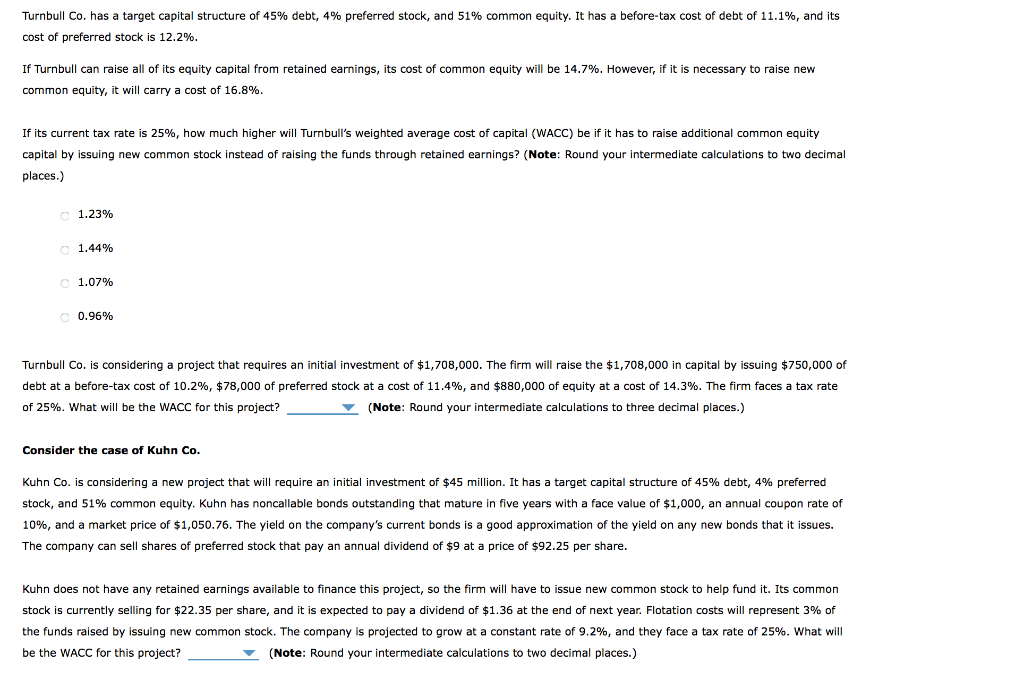Turnbull Co. has a target capital structure of 45% debt, 4% preferred stock, and 51% common equity. It has a before-tax cost of debt of 11.1%, and its cost of preferred stock is 12.2% If Turnbull can raise all of its equity capital from retained earnings, its cost of common equity will be 14.7%. However, if it is necessary to raise new common equity, it will carry a cost of 16.8%. If its current tax rate is 25%, how much...

• ### 6. Solving for the WACC The WACC is used as the discount rate to evaluate various...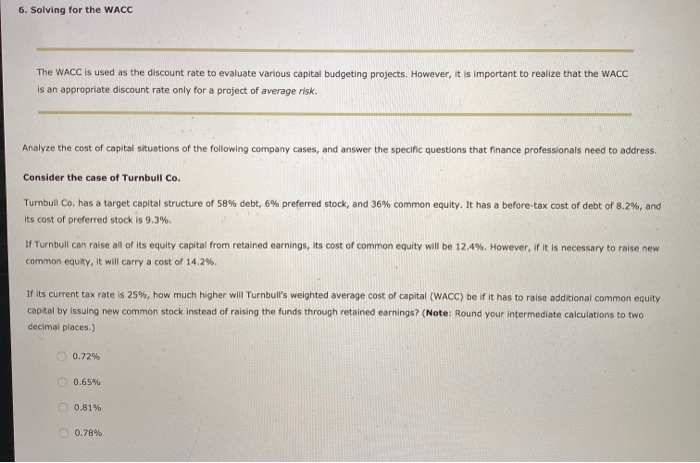6. Solving for the WACC The WACC is used as the discount rate to evaluate various capital budgeting projects. However, it is important to realize that the WACC is an appropriate discount rate only for a project of average risk. Analyze the cost of capital situations of the following company cases, and answer the specific questions that finance professionals need to address. Consider the case of Turnbull Co. Turnbull Co. has a target capital structure of 589% debt, 6 %...